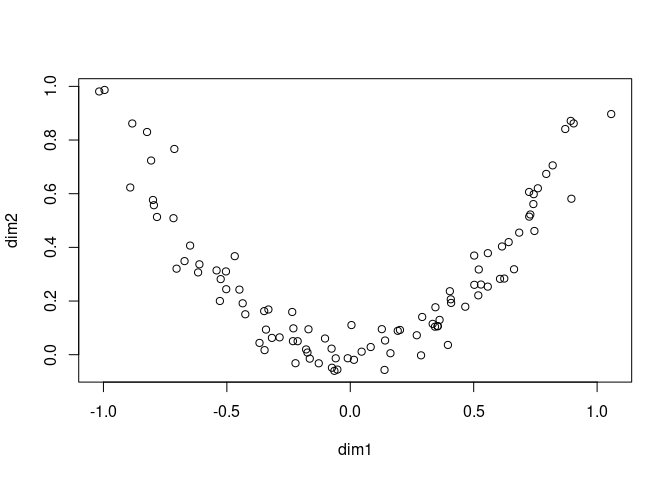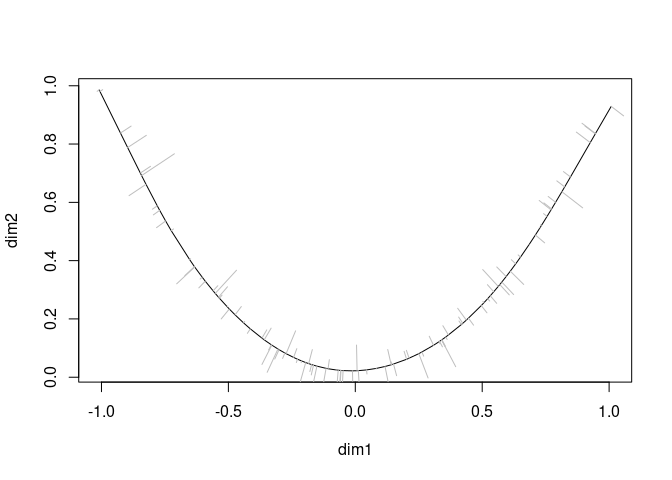# princurve

Fitting a principal curve to a data matrix in arbitrary dimensions. A principal curve is a smooth curve passing through the middle of a multidimensional dataset. This package is an R/C++ reimplementation of the S/Fortran code provided by Trevor Hastie, with multiple performance tweaks.

## Example

Usage of princurve is demonstrated with a toy dataset.

``````t <- runif(100, -1, 1)
x <- cbind(t, t ^ 2) + rnorm(200, sd = 0.05)
colnames(x) <- c("dim1", "dim2")

plot(x)``````A principal curve can be fit to the data as follows:

``````library(princurve)
fit <- principal_curve(x)
plot(fit); whiskers(x, fit\$s, col = "gray")``````See `?principal_curve` for more information on how to use the `princurve` package.

## Latest changes

Check out `news(package = "princurve")` or NEWS.md for a full list of changes.

### Recent changes in princurve 2.1.6 (2021-01-17)

• BUG FIX `project_to_curve()`: Return error message when `x` or `s` contain insufficient rows.

• BUG FIX unit tests: Switch from `svg()` to `pdf()` as support for `svg()` might be optional.

### Recent changes in princurve 2.1.5 (2020-08-13)

• BUG FIX `project_to_curve()`: Fix pass-by-reference bug, issue #33. Thanks to @szcf-weiya for detecting and fixing this bug!

Hastie, T. and Stuetzle, W., Principal Curves, JASA, Vol. 84, No. 406 (Jun., 1989), pp. 502-516, DOI: 10.2307/2289936 (PDF)